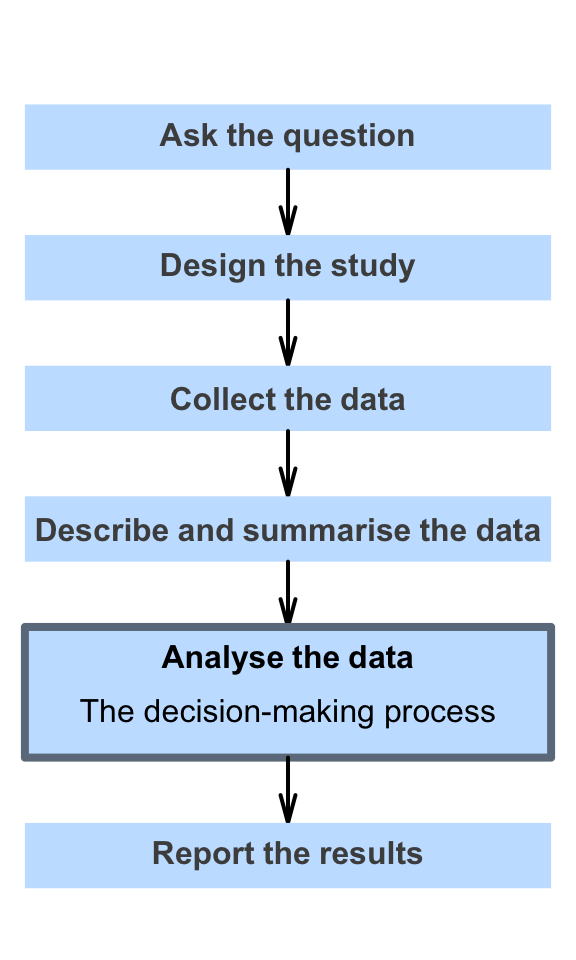# 15 Making decisions: An introduction

So far, you have learnt to ask a RQ, identify different ways of obtaining data, design the study, collect the data describe the data, and summarise data graphically and numerically.

In this chapter, you will learn how decisions are made in science, so we can answer RQs. You will learn to:

• explain the two broad reasons why differences are seen between sample statistics.
• explain how decisions are made in research.